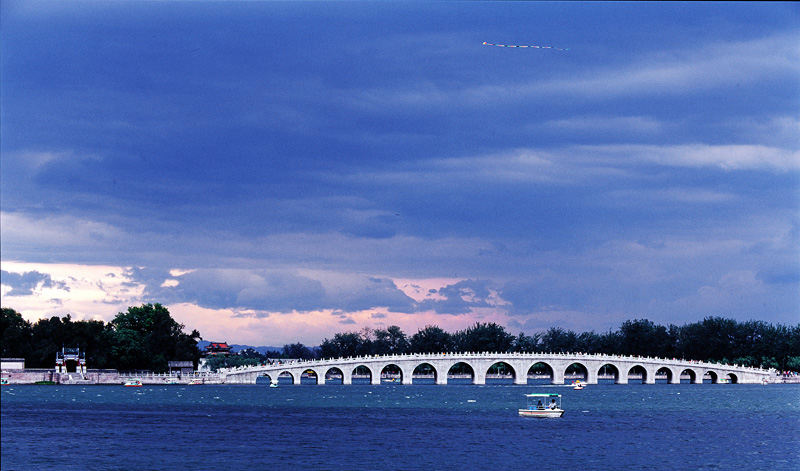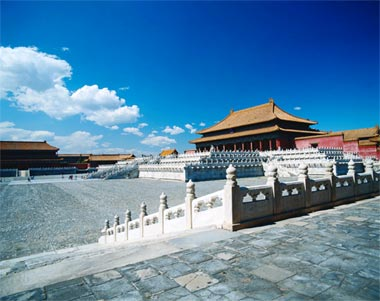# Beijing, June 09- 12, 2008The goal of this conference is to survey recent advances on a wide range of topics of current interest in nonlinear waves and related phenomena including: integrable and nonintegrable equations, physical applications such as optics, Bose-Einstein condensates and fluid dynamics, and numerical computations. The conference will provide a forum for mathematicians and physicists worldwide to present their latest results and a means to discuss their recent research with each other. Themes of this conference include(but are not limited to): Continuous and discrete integrable equations Algebraic methods of integrable equations New types of solutions in integrable equations Riemann-Hilbert problems Semi-classical limits of integrable equations Solitary wave interactions and collisions in non-integrable systems Stability theory of solitary waves Numerical computations of nonlinear-wave problems Nonlinear waves in periodic and inhomogeneous media Nonlinear optics Fluid dynamics Bose-Einstein condensation Wave phenomena in the atmosphere and the ocean